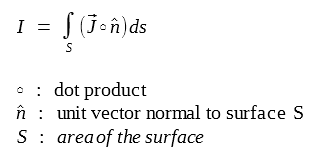# A Plain Explanation of Maxwell's Equations

## Physical Meanings of Maxwell's Equations

Maxwell's Equations are composed of four equations with each one describes one phenomenon respectively.

Maxwell didn't invent all these equations, but rather he combined the four equations made by Gauss (also Coulomb), Faraday, and Ampere.

But Maxwell added one piece of information into Ampere's law (the 4th equation) - Displacement Current, which makes the equation complete.

1. Gauss's law for static electric fields
2. Gauss's law for static magnetic fields
3. Faraday's law which says a changing magnetic field (changing with time) produces an electric field
4. Ampere-Maxwell's law which says a changing electric field (changing with time) produces a magnetic field

The combination of equations 3 and 4 can explain electromagnetic wave (such as light) which can propagate on its own.

The combination says that a changing magnetic field produces a changing electric field, and this changing electric field produces another changing magnetic field. Thus the cycle continues and an electromagnetic wave is made and propagates through the space.

## Mathematical Representations

Maxwell's equations can be expressed in two forms:

• Integral Equations Form
• Differential Equations Form
Equation Name Integral Equations Form

Gauss's Law
for Static Electric Fields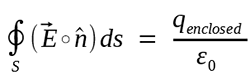• Electric charge q produces an electric field E
• The electric field flux passing through any closed surface is proportional to the total charge contained within that surface

Gauss's Law
for Static Magnetic Fields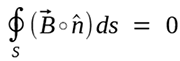• The total magnetic flux passing through any closed surface is 0.
• The assumption that there are no magnetic monopoles.
• There are no magnetic flow sources, and the magnetic flux lines always close upon themselves.
• Also called the law of conservation of magnetic flux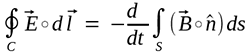• Changing magnetic flux through a surface induces an electromotive force (EMF) in any boundary path of that surface.
• A changing magnetic field induces a circulating electric field.
• The voltage accumulated around a closed circuit is proportional to the time rate of change of the magnetic flux it encloses.

Ampere's Law
(with Maxwell's Addition of Displacement Current)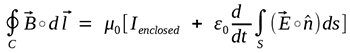• An electric current I or a changing electric flux through a surface produces a circulating magnetic field around any path that bounds that surface.
• Electric currents and changes in electric fields are proportional to the magnetic fields circulating about the areas where they accumulate.

Equation Name

Differential Equations Form

(Point Functions)

Gauss's Law
for Static Electric Fields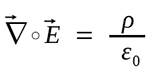The electric field produced by electric charge diverges from positive charge and converges upon negative charge.

Gauss's Law
for Static Magnetic Fields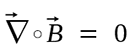• The divergence of the magnetic field at any point is zero.
• The assumption that there are no magnetic monopoles.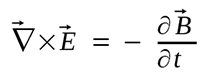A circulating electric field is produced by a magnetic field that changes with time.

Ampere's Law
(with Maxwell's Addition of Displacement Current)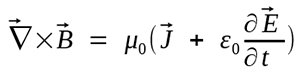A circulating magnetic field is produced by an electric current and by an electric field that changes with time.

## Integral Forms

### 1. Gauss's Law for Static Electric Fields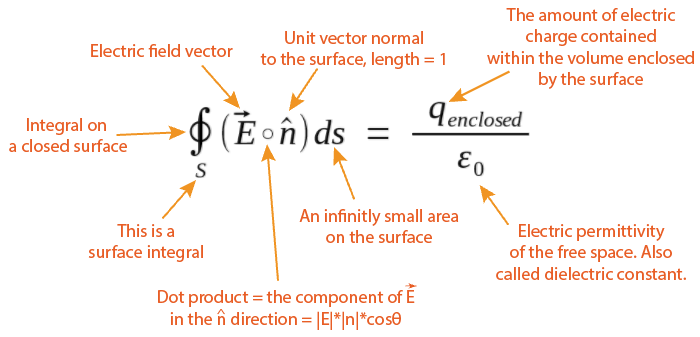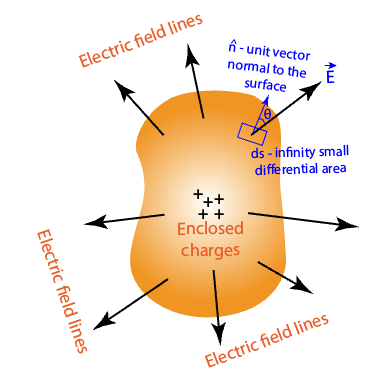Concepts Meaning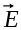Electric Field

• The electric force (newtons) experienced by one coulomb of test charge in the field.
• Unit is newtons/coulomb or volts/meter.

Dot Product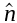Unit Normal Vector

• n is a vector with length of one pointing in the direction perpendicular to the surface.
• At any point on the closed surface we can find a different nThe Component of E Normal to a Surface

• This represents the component of the electric field vector that is perpendicular to the surface under consideration
• Used to calculate the electric flux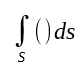Surface Integral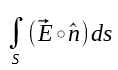The Flux of Electric Field E Over a Surface S

• The analog of fluid flow is very useful for understanding the meaning of the "flux" of a vector field
• Flux of a vector field over a surface is the "amount" of that field that "flows" through the surface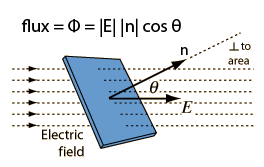The Electric Flux Through a Closed Surface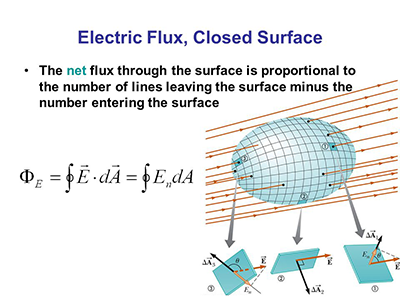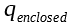The Enclosed Elctric Charge within the Closed Surface

Because any charge located outside the closed surface produces an equal amount of inward (negative) flux and outward (positive) flux, so the net flux is only determined by the charges within the closed surface.

If the total enclosed charge is positive, then we have a positive (outward) net flux. If the total enclosed charge is negative, then we have a negative net flux (inward).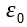The Permittivity of Free Space

The permittivity of a material determines its response to an applied electric field

In dielectric materials, charges do not move freely, but may be slightly displaced from their equilibrium position (called polarization) because the electric field.

The permittivity of a medium is a fundamental parameter in determing the speed with which an electromagnetic wave propagates through that medium.

ε0 = 8.8541878176 x 10-12 coulombs/(volt-meter)

### 2. Gauss's Law for Static Magnetic Fields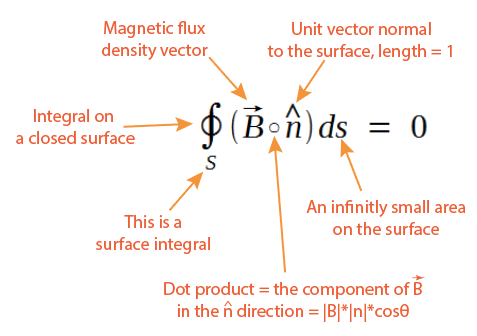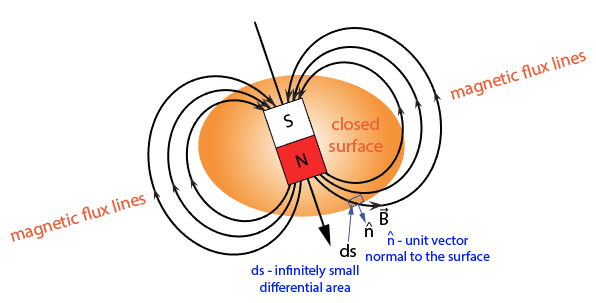Concepts Meaning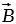Magnetic Flux Density

Also called magnetic field. The magnetic field is defined using the magnetic force experienced by a moving charged particle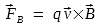• FB is the magnetic force
• q is the test particle's charge
• v is the particle's velocity
• B is the magnetic flux density
• x is the cross product of two vectors
• the magnitude of B can be written as: (θ is the angle between v and B)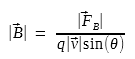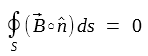There are no magnetic monopoles

• The net outward magnetic flux through any closed surface is always zero
• There are no magnetic flow sources such as magnetic monopoles
• Magnetic flux lines always close upon themselves, forming continuous loops
• The traditional designation of north and south poles in a permanent bar magnet does not mean that an isolated positive magnetic charge exists at the north pole and a corresponding amount of isolated negative magnetic charge exists at the south pole.
• If this magnetic bar is cut into two segments, new south and north poles appear. Magnetic poles cannot be isolated.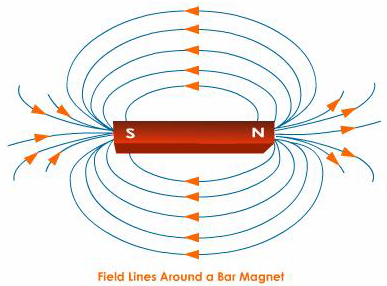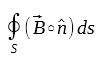The Magnetic Flux Through a Closed Surface

Like the electric flux, the magnetic flux through a surface is the "amount" of magnetic field "flowing" through the surface.

For a closed surface, the surface penetration is a two-way street: the outward flux is positive, and the inward flux is negative. The net flux is the sum of outward flux and inward flux.

### 3. Faraday's Law of Induction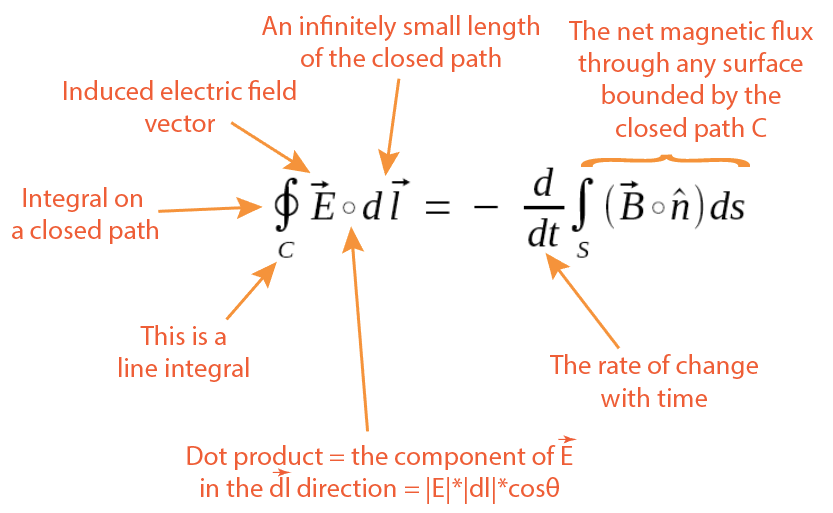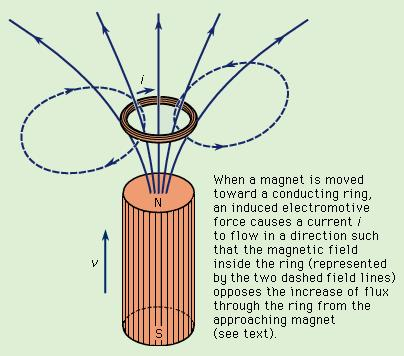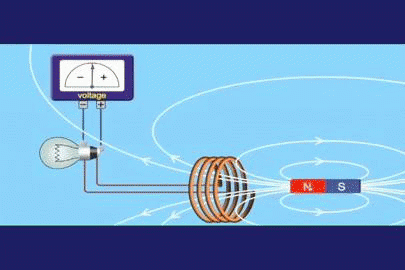Concepts MeaningThe Inducecd Electric Field

• This is the induced electric field by changing magnetic flux
• This induced electric field's structure is different from the electrostatic field produced by charged particles as shown below
• Charge-based electric fields originate on positive charge and terminate on negative charge
• Induced electric field loop back on themselves, with no point of origination or termination
• The induced electric field drives an electric current which produces magnetic flux to oppose the change in flux due to the changing magnetic field (trying to keep the magnetic flux stable)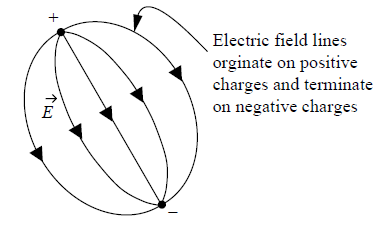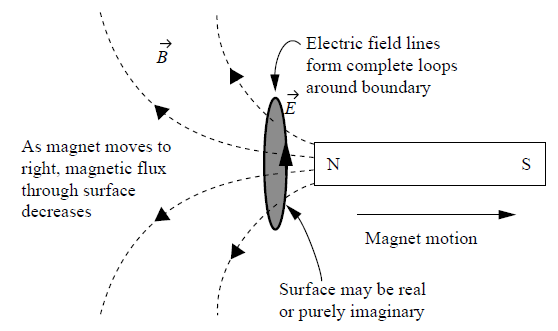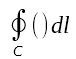The Line Integral Over a Closed Path C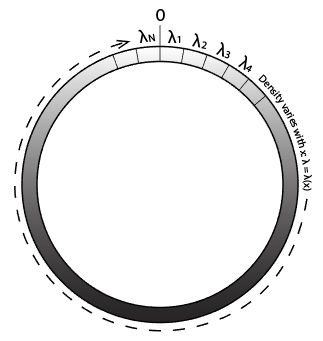• The wire aboves has varying density λ(x) and we want to know its total mass
• Imagine dividing the wire into a series of very short segments (N pieces) such that the density λi is approximately constant
• The total mass of the wire is then the sum of all the segment masses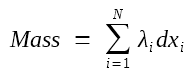If we make the pieces infinitely small (N -> ∞) , then the summation becomes a line integral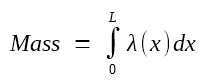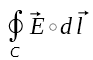The Induced Electric Field Circulation

• Induced electric fields form closed loops, thus they can drive charged particles around continuous circuits and produce an electric current
• This circulation of electric field is called "electromotive force" or EMF
• This is not a force, but rather a force per unit charge integrated over the length of the circuit
• Physical this means the work done by the induced electric field in moving a unit charge around that path.
• In other words, it is the energy given to one coulomb of charge as it moves around the circuit.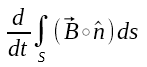The Rate of Change of Magnetic Flux

• The integration is simply the magnetic flux through the surface bounded by the closed path C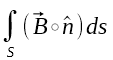• This magnetic flux is not zero! This is the magnetic flux through a open surface, not a closed surface. Don't confuse this with Gauss's law for static magnetic field.
• Then take a direvative over time (t), this is the rate of change of the magnetic flux• The magnetic flux can be changed by three means:
• The magnitude of B can change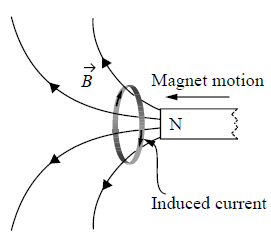• The angle between B and the surface normal can change (cosθ by the dot product)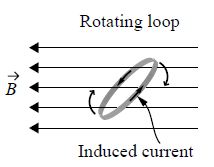• The area of the surface can change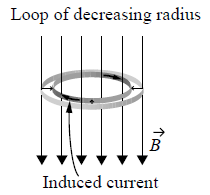### 4. Ampere-Maxwell's Law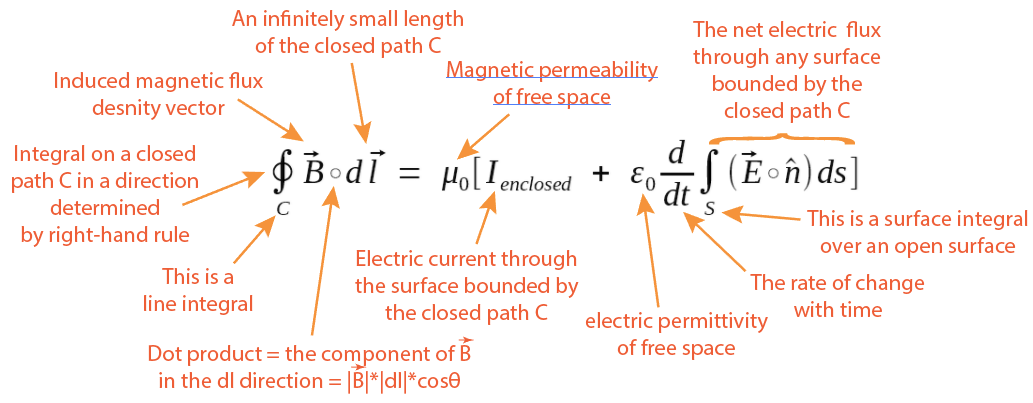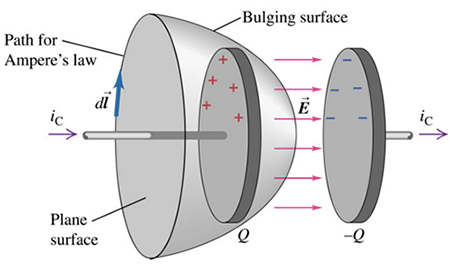Concepts Meaning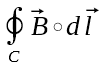The Induced Magnetic Field Circulation

An electric current or a changing electric flux through a surface produces a circulating magnetic field around any path that bounds that surface.

The current and the induced magnetic field follow the right-hand rule as shown below.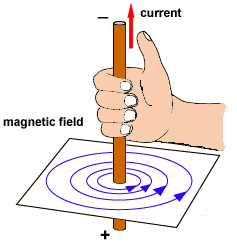This magnetic field circulation is calculated by doing a line integral of the dot product of B and dl (an infinitely small differential length of the path) on the closed path C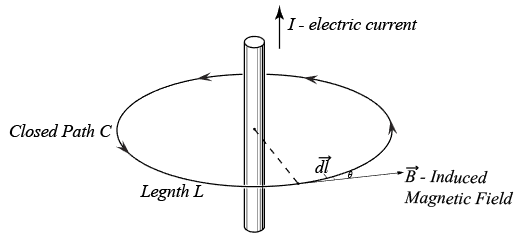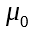The Permeability of Free Space

• The magnetic permeability determines a material's response to an applied magnetic field.
• Here is the permeability of free space ("vacuum permeability")
 μ0 = 4π x 10-7 Vs/Am (volt-seconds per ampere-meter)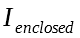The Enclosed Electric Current

• "Enclosed" means that this current penetrates through any surface which is bounded by the closed path C
• We must consider the direction of the electric current relative to the direction of magnetic field B's line integration.
• The right-hand rule determines whether a current is counted as positive or negative
 This electric current is considered as being enclosed since it penetrates any surface bounded by the closed path C1.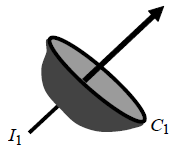This electric current is considered as not being enclosed because it doesn't penetrate one of the surfaces bounded by the closed path C2.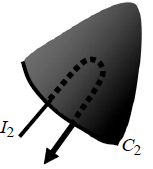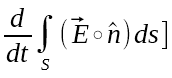The Rate of Change of Electric Flux (Displacement Current)

This term is the electric flux analog of the changing magnetic flux term in Faraday's law of induction as mentioned above.

Faraday's law of induction says that a changing magnetic flux through any surface induces a circulating electric field along a boundary path.

This part was added by Maxwell into the original Ampere's equation. It says that a changing electric flux through a surface should induce a circulating magnetic field around a boundary of that surface.

This term solved the capacitor charging/discharging paradox which cannot be counted as an ecnlosed electric current.

In the following figure, should ic be considered an enclosed current?

No. Instead we should count the electric field flux change between the plates of the capacitor (Q and -Q). This electric flux change happens when the capacitor is charging or discharging.This electric flux change is calculated by integrating electric field E over the bulging surface which is bounded by the closed path C and then take the direct direvative over time (t).

## Differential Forms

### 1. Gauss's Law for Static Electric Field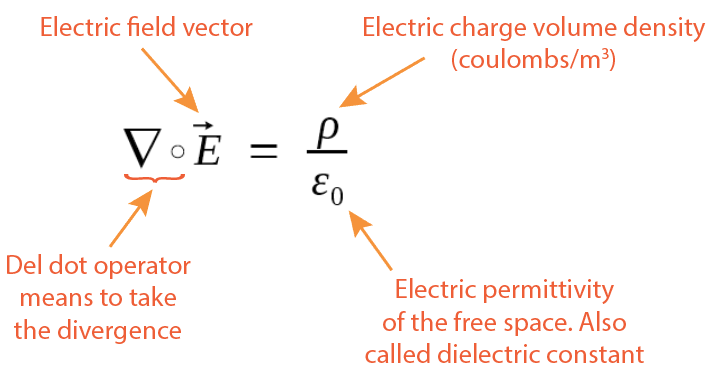In an static electric field, the divergence at one point equals to the electric charge volume density ρ at that point divided by ε0. So if you know the electric charge distribution, you can calculuate the electric field produced.

Concepts Meaning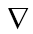Nabla - the Del (Gradient) Operator

The nabla symbol is a mathematical operator (just like the square root symbol). It means to take derivatives of the quantity following it which is the quantity's gradient.

In Cartesian coordinate system, the Del operator is defined as: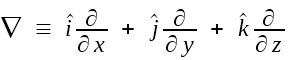where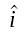is the unit vector in the x axis,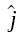is the unit vector in y axis, and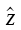is the unit vector in z axis.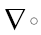Del Dot - The Divergence Operator

• Del dot operator takes the divergence at a single point in the field.
• Divergence measures the tendency at one point that the field vectors "carries" more material away from it than is brought toward it
• Points of positive divergence are "sources"
• Points of negative diverngece are "sinks"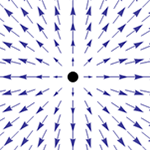Positive divergence at the origin point (a source)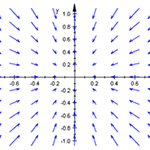Negative divergence at the orgin point (a sink)

Divergence is a scalar quantity (not a vector). But the field that it operates on is a vector.

Flux is defined over an area, while divergence is defined at individual points, don't confuse them.

Divergence is the limit of flux through an infinitesimal surface surrounding the point as defined below.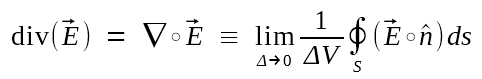ρ

Charge volume density at one point, its unit is coulombs / meter3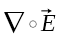The Divergence of the Electric Field

In Cartesian coordinate, the electric field vector can be decomposed into three components.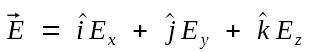According to the definition of the del dot operator, we then have: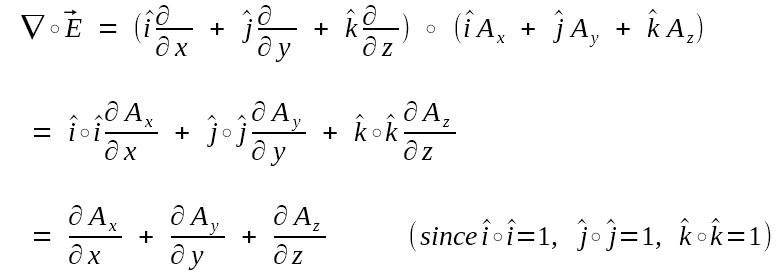Thus, the divergence of an electric field is the sum of

• The change of its x-component along the x axis
• The change of its y-component along the y-axis
• The change of its z-component along the z-axis

### 2. Gauss's Law for Static Magnetic Fields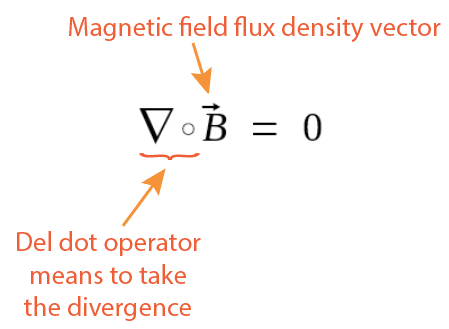Divergence is a measurement of if there are more "flowing away" from a point than toward that point. It there are more "flowing away", it is a "source", if there are more "flowing in" then it is a sink. However, since we have never discovered magnetic monopoles (south pole only or north pole only), there are always same amount of "flowing out" and "flowing in" at a point in magnetic fields. This statment stresses that there are no magnetic monopoles.

### 3. Faraday's Law of Induction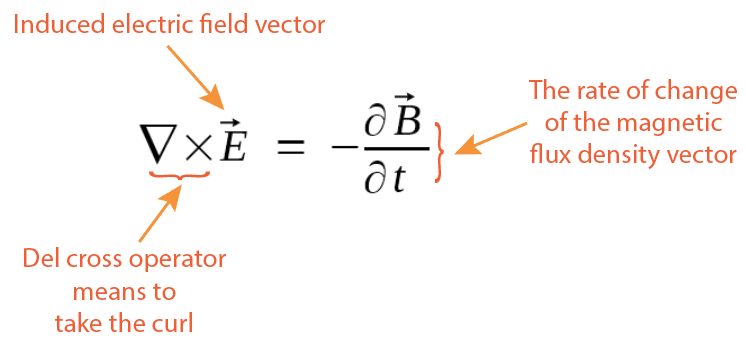The physical meaning is that a changing magnetic field produces a circulating electric field. This is a point function, it applies to every point in space, whether it be in free space or in a material medium. The electric field intensity in a region of time-varying magnetic flux density is therefore nonconservative and cannot be expressed as the gradient of a scalar potential.

Concepts Meaning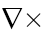Del Cross - the Curl Operator

You get the curl of the point of interest in a vector field A by following the steps below:

1. Make a small circle around the point of interest, call it the closed path C
2. Calculate the circulation by doing a line integral of A around path C. This line integral is done by always keeping the inside of the circle at the left side of the path.
3. Divide this circulation by the area of the circle
4. Make the circle infinitesimal small and the limit is the curl
5. The direction of the curl is then the normal direction of the surface for which the circulation is a maximum
6. The positive-curl direction is determined by the right-hand rule: if you curl the fingers of your right hand along the circulation, you thumb points in the direction of positive curl.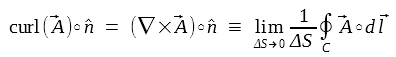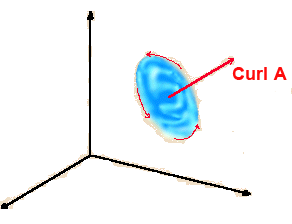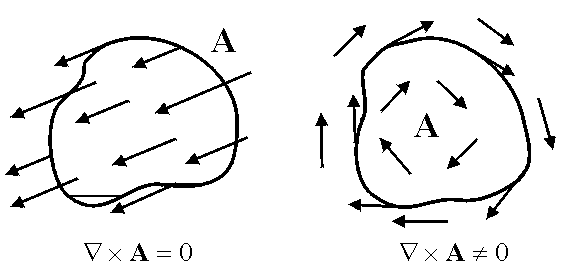The curl of a vector field is a point function and it measures how how much of the vector A swirls around the point in question. The curl of a vector field is another vector field, just like any cross product.

In Cartisian coordinates, curl is calculated by doing partial derivates expressed as a determinant: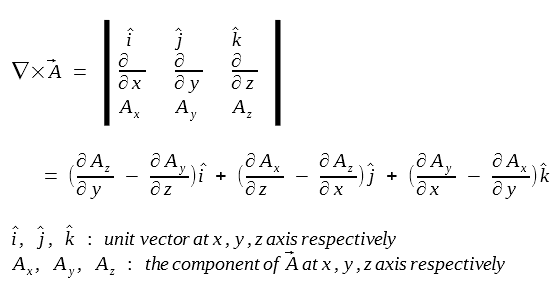Each component of the curl of A indicates the tendency of the field to rotate around the respective axis x, y, z.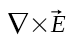Curl of the Electric Field

Charge Based Fields:

Charge based electric fields diverge from the points of positive charge and converge to points of negative charge. This type of fields do not circulate back on themselves and their curls are zero at every point.

Induced Fields:

Induced electric fields by changing magnetic fields are very different from charge based electric fields.

Induced fields circulate back onto themselves and have no origination or termination points. Thus induced electric fields have curl. The faster the magnetic field changes, the larger the curl of the induced electric field.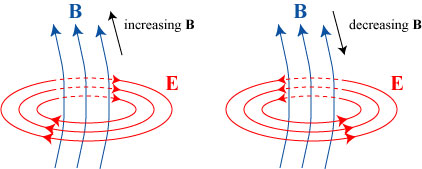### 4. Ampere-Maxwell's Law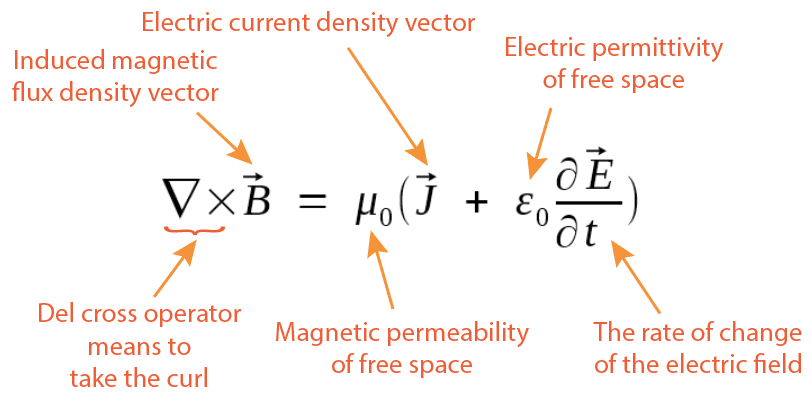In an static electric field, the divergence at one point equals to the electric charge volume density ρ at that point divided by ε0. The physical meaning is: A circulating magnetic field is produced by an electric current and/or by an electric field that changes with time.

Concepts Meaning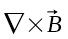The Curl of the Induced Magnetic Field

All magnetic fields circulate back upon themselves, and it curl is non-zero at exact the locations through which an electric current is flowing or an electric field is changing.

Just because the field appears to curve doesn't mean that the curl is non-zero everywhere! This is a common mistake. Look at the picture below.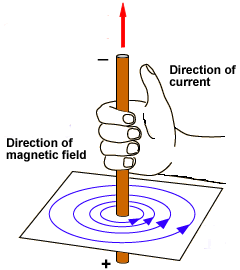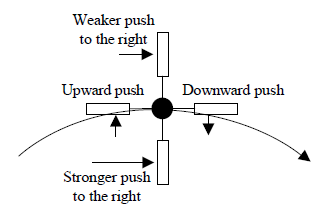It looks like the paddlewheel would rotate clockwise owing to the curvature of the field. However, the downward curvature of the field is offset by the weakening of the field with the distance from the center of curvature.

The upward-downward push is exactly compensated by the weaker-stronger push and the paddlewheel does not turn. The curl is zero at this location even though the field lines are curved!

The magnetic field may be curved at many locations, but only at points where electric current is flowing or the electric flux is changing that the curl is non-zero.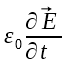The Displacement Current Density

Displacement current density has the units of electric current density (Amps/meters2), and it has an associated magnetic field just as actual current do.

However, displacement current doesn't represent an actual current of moving charges, but a time-varying electric field, it is the rate of change of electric field with time.

In dielectric materials (as opposed to vacuum), displacement current also has a contribution from slight displacement of bounded charges (which is called dielectric polarization).

The displacement current term was a crucial addition to Ampere's Circuital Law that completed Maxwell's equations and units electricity, magnetism and optics into one single unified electromagnetics theory.

Adding this term (changing electric field as a source of the magnetic field) extended Ampere's law to time-dependent fields by eliminating the inconsistency with the principle of conservation of charge.

The key concept here is that a changing electric field produces a changing magnetic field even when no charges are present and no physical current flows. Through this mechanism, electromagnetic waves (optical waves included) can propagate through a perfect vacuum, as changing magnetic fields induce electric fields, and changing electric fields induce magnetic fields.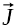The Conduction Electric Current Density

The electric current density is defined as the vector current flowing through a unit cross-secional area perpendicular to the direction of the current. Thus it has a unit of amperes per sqare meter (A/m2).

The electric current density in Ampere-Maxwell law includes all currents, including the bound current density in magnetic materials.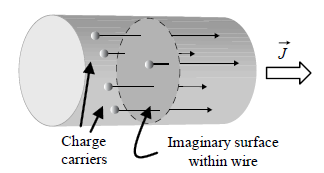If the number density of charge carriers are n and the charge per carrier is q, then the amount of charge passing through a unit area perpendicular to the flow per second is: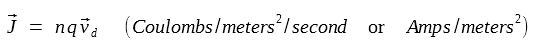Total current I vs current density J:

If J is uniform over a surface S: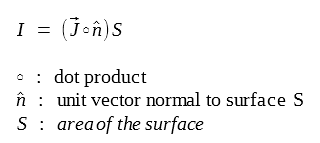If J is not uniform: